Home > CCA2 > Chapter 8 > Lesson 8.3.2 > Problem8-153

8-153.
1. Sketch a graph of each of the following trigonometric functions. 8-153 HW eTool (Desmos). Homework Help ✎

1. y = sin(0.5(x π))

2. y = 10sin(3x) − 2

3. y = 5cos(x +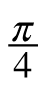)

4. y = cos(2(x))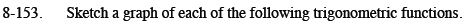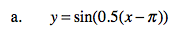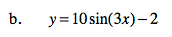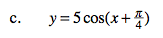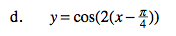Use the eTool below to help you with this problem.
Click on the link at right for the full eTool version: CCA2 8-153 HW eTool